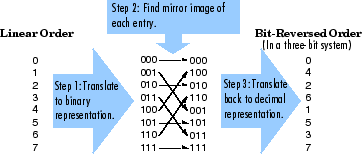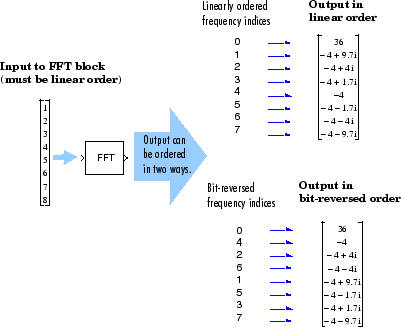Main Content

## Linear and Bit-Reversed Output Order

### FFT and IFFT Blocks Data Order

The FFT block enables you to output the frequency indices in linear or bit-reversed order. Because linear ordering of the frequency indices requires a bit-reversal operation, the FFT block may run more quickly when the output frequencies are in bit-reversed order.

The input to the IFFT block can be in linear or bit-reversed order. Therefore, you do not have to alter the ordering of your data before transforming it back into the time domain. However, the IFFT block may run more quickly when the input is provided in bit-reversed order.

### Find the Bit-Reversed Order of Your Frequency Indices

Two numbers are bit-reversed values of each other when the binary representation of one is the mirror image of the binary representation of the other. For example, in a three-bit system, one and four are bit-reversed values of each other, since the three-bit binary representation of one, 001, is the mirror image of the three-bit binary representation of four, 100. In the diagram below, the frequency indices are in linear order. To put them in bit-reversed order

1. Translate the indices into their binary representation with the minimum number of bits. In this example, the minimum number of bits is three because the binary representation of 7 is 111.

2. Find the mirror image of each binary entry, and write it beside the original binary representation.

3. Translate the indices back to their decimal representation.

The frequency indices are now in bit-reversed order.The next diagram illustrates the linear and bit-reversed outputs of the FFT block. The output values are the same, but they appear in different order.## Support